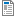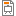Lesson Plan: Transformations in Math and Art
Student Learning Objectives
• Create a symmetrical design using only squares, rectangles, and triangles in one quadrant of the coordinate plane.
• Transform the shapes to the other quadrants to form a symmetrical design.
Math

CCSS.Math.Content.8.G.1, CCSS.Math.Content.8.G.2, CCSS.Math.Content.8.G.3

Visual Arts

8.CR.1.2

8.V.1.2

Written by Tonya Buff, Math Teacher
Essential Question: How can I use math concepts in art making?
Abstract: Students will observe and describe examples of rotations, translations, and reflections in various works of art. Students will then create a work of art using these mathematical concepts. Finally, students will describe the transformations from their artwork using ordered pairs.

SUBJECT AREAS

CONCEPTS

1. Students view various works of art from the Museum that are examples of the transformations (PowerPoint).  For each work of art, teacher asks the following:
• What do you see?
• What does this work of art make you wonder?

Teacher then asks students if the work of art contains an example of slide, flip, or turn, as directed in the PowerPoint presentation. After the presentation, the teacher reviews the elements of art and principles of design and asks the following:

• How have the artists applied the principles of design in these works of art?
• Why might the artists have created works of art that feature symmetry?
• What might the artists need to know about math to create these works of art?
• How might you use the principles of design in creating your own work of art that features slide, flip, or turn?
1. Students are given a description of the project and examples (PowerPoint).
2. Students find the center of their graph paper and create a coordinate plane.
3. Students draw a diagonal line through quadrants to form an X.
4. Students plan then construct their design in one half of one quadrant then transform the design to the other half.
5. Students replicate the design to the other quadrants.
6. Students color the design using symmetrical color scheme.
7. Students record the original coordinates of at least five shapes, describe the transformation, and then record the new coordinates of the figures.
8. Students complete a self-assessment of their work using a rubric.
9. Teacher assesses the work using a rubric.
• Student handout will be used to assess students’ ability to describe the effect of dilations, translations, rotations, and reflections on two-dimensional figures using coordinates.
• Rubric will be used to assess student planning and creation of a work of art that demonstrates understanding of transformation and congruence.
• Student self-assessment will be used to assess students’ ability to critique art based on persobe used to assess student planning and creation of a work of art that demonstrates understanding of transformation and congruence.
Vocabulary

coordinate plane
ordered pair
x-coordinate
y-coordinate
symmetry
transformation
translation (slide)
rotation (turn)
reflection (flip)

Materials
• Slides of works of art
• Power Point
• Graph paper
• Pencils
• Rulers
• Crayons or colored pencils
• Notebook paper
• Rubric

Lesson Plan DocumentTransformations in Art and Math.docxTransformations in Art Student Rubric.docxTransformations in Math and Art Rubric for Teacher Scoring.docxTransformations.ppt